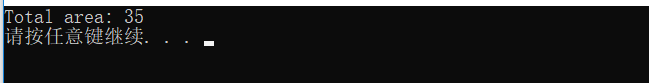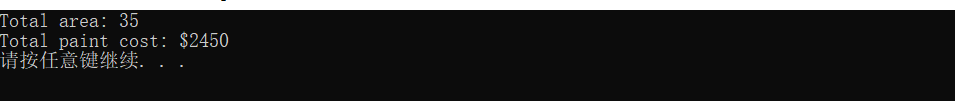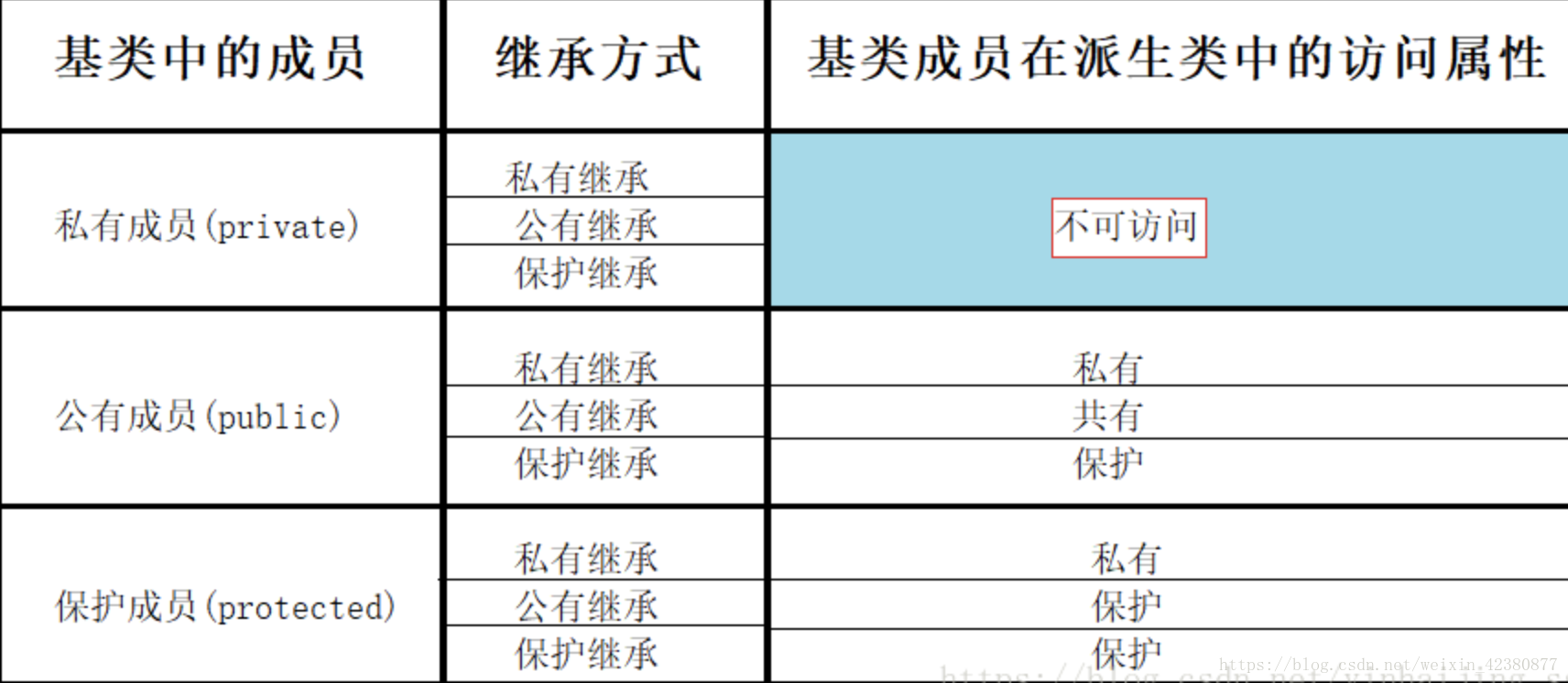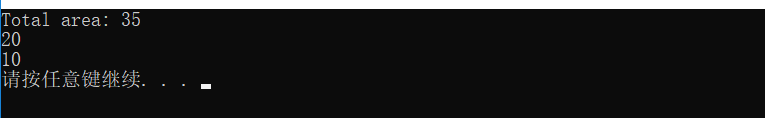# ·类的继承

class 派生类名（子类名）：继承方式   基类名
{
派生类成员声明；
};

#include <iostream>

using namespace std;

// 基类
class Shape
{
public:
void setWidth(int w)
{
width = w;
}
void setHeight(int h)
{
height = h;
}
protected:
int width;
int height;
};

// 派生类
class Rectangle: public Shape
{
public:
int getArea()
{
return (width * height);
}
};

int main(void)
{
Rectangle Rect;           //声明一个对象

Rect.setWidth(5);         //赋值
Rect.setHeight(7);

// 输出对象的面积
cout << "Total area: " << Rect.getArea() << endl;
system("pause");
return 0;
}多继承时：

class 派生类名：继承方式  基类名1，继承方式  基类名2，······，继承方式  基类名n
{
派生类成员声明；
};

#include <iostream>

using namespace std;

// 基类 Shape
class Shape
{
public:
void setWidth(int w)
{
width = w;
}
void setHeight(int h)
{
height = h;
}
protected:
int width;
int height;
};

// 基类 PaintCost
class PaintCost
{
public:
int getCost(int area)
{
return area * 70;
}
};

// 派生类
class Rectangle : public Shape, public PaintCost         //继承多个类
{
public:
int getArea()
{
return (width * height);
}
};

int main(void)
{
Rectangle Rect;                   //对象
int area;

Rect.setWidth(5);
Rect.setHeight(7);

area = Rect.getArea();

// 输出对象的面积
cout << "Total area: " << Rect.getArea() << endl;

// 输出总花费
cout << "Total paint cost: \$" << Rect.getCost(area) << endl;
system("pause");
return 0;
}继承分为3类：1.公有继承（public ）：基类的公有成员和保护成员的访问属性在派生类中不变，而基类的私有成员不可直接访问

2.私有继承（private）：基类中的公有成员和保护成员都以私有成员身份出现在派生类中，而基类的私有成员在派生类中不可直接访问

3.保护继承（protected）：基类的共有成员和保护成员都以保护成员的身份出现在派生类中，而基类的私有成员不可直接访问# ·类的派生

2.改造基类成员

3.添加新的成员

1.访问子类同名成员 直接访问即可

2.访问父类同名成员 需要加作用域

#include <iostream>

using namespace std;

// 基类
class Shape
{
public:
void setWidth(int w)
{
width = w;
}
void setHeight(int h)
{
height = h;
}
protected:
int width;
int height;
int a = 10;
};

// 派生类
class Rectangle : public Shape
{
public:
int getArea()
{
return (width * height);
}
void show()
{
cout << a << endl;                      //访问子类同名成员
cout << Shape::a << endl;           //访问父类同名成员
}
private:
int a = 20;
};

int main(void)
{
Rectangle Rect;           //声明一个对象
Rect.setWidth(5);         //赋值
Rect.setHeight(7);

// 输出对象的面积
cout << "Total area: " << Rect.getArea() << endl;
Rect.show();
system("pause");
return 0;
}posted @ 2019-10-13 19:03  Zzzxy  阅读(175)  评论(0编辑  收藏  举报